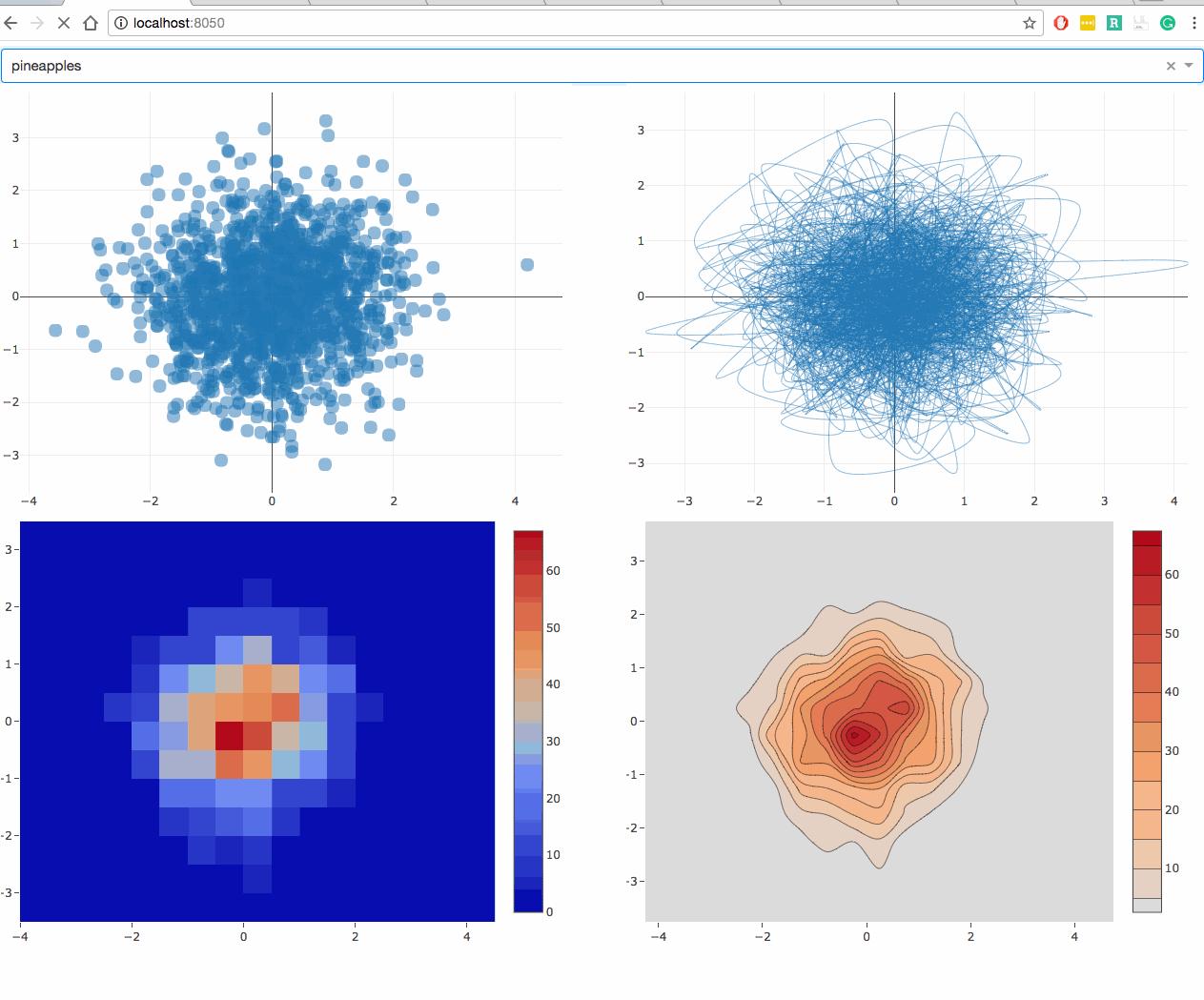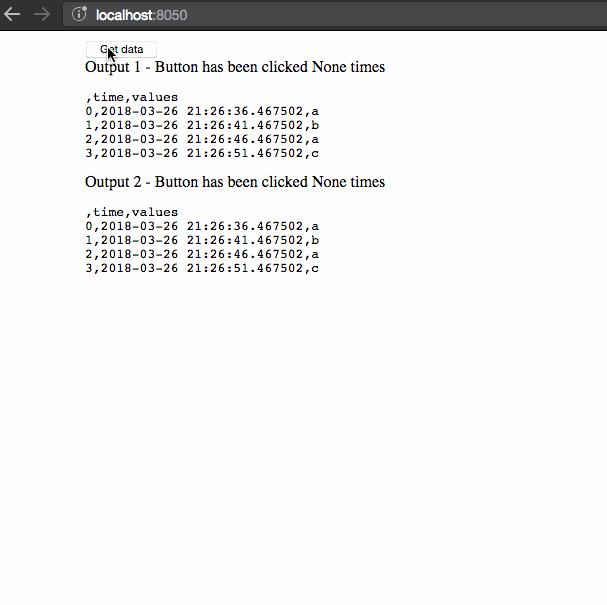# 【Python百日基础系列】Day29 - Dash回调间共享数据

wuchangjian2021-11-15 18:36:19编程学习

### 文章目录

• 注意：本节内容需要Redis服务器支持，我用不大上，所以没有配置。
• 一、存储共享数据
• 二、在浏览器中存储数据 dcc.Store
• 三、缓存和信令
• 3.1 代码
• 3.2 页面效果
• 3.3 注意事项
• 四、服务器上基于用户的会话数据
• 4.1 代码
• 4.2 页面效果
• 4.3 注意事项

### 注意：本节内容需要Redis服务器支持，我用不大上，所以没有配置。

dcc.Graph使用开源plotly.js JavaScript 图形库呈现交互式数据可视化。Plotly.js 支持超过 35 种图表类型，并以矢量质量 SVG 和高性能 WebGL 呈现图表。

# 一、存储共享数据

• 在用户的浏览器会话中，使用dcc.Store
• 在磁盘上（例如在文件或数据库中）
• 在跨进程和服务器（例如 Redis 数据库）共享的服务器端内存 (RAM) 中。为此，Dash Enterprise包含板载的一键式 Redis 数据库。

# 二、在浏览器中存储数据 dcc.Store

1. 数据必须转换为字符串，如 JSON 或 base64 编码的二进制数据以进行存储
2. 以这种方式缓存的数据将仅在用户的当前会话中可用。
• 如果您打开一个新的浏览器窗口，应用程序的回调将始终重新计算数据。数据仅在同一会话内的回调之间缓存。
• 此方法不会增加应用程序的内存占用。
• 网络流量可能会产生成本。如果您在回调之间共享 10MB 的数据，那么该数据将在每个回调之间通过网络传输。
• 如果网络成本太高，则预先计算聚合并传输它们。您的应用程序可能不会显示 10MB 的数据，它只会显示它的子集或聚合。
下面的示例显示了您可以利用的一种常用方法dcc.Store：如果处理数据集需要很长时间并且不同的输出使用此数据集，dcc.Store则可用于将处理后的数据存储为中间值，然后可将其用作多个输入回调以生成不同的输出。这样，昂贵的数据处理步骤仅在一个回调中执行一次，而不是在每个回调中多次重复相同的昂贵计算。
1. 如果数据很大，通过网络发送计算数据可能会很昂贵。在某些情况下，将此数据序列化为 JSON 的成本也很高。
2. 在许多情况下，您的应用程序只会显示处理数据的子集或聚合。在这些情况下，您可以在数据处理回调中预先计算聚合并将这些聚合传输到剩余的回调。

# 三、缓存和信令

1. 通过 Flask-Cache 使用 Redis 将“全局变量”存储在服务器端的数据库中。该数据通过函数 ( global_store())访问，该函数的输出由其输入参数缓存和键控。
2. dcc.Store当昂贵的计算完成时，使用该解决方案向其他回调发送信号。
3. 请注意，除了 Redis，您还可以将其保存到文件系统。
4. 这种“signaling”是高性能的，因为它允许昂贵的计算只占用一个进程并执行一次。如果没有这种类型的信号，每个回调最终可能会并行计算昂贵的计算，锁定四个进程而不是一个。

## 3.1 代码

``````import os
import copy
import time
import datetime

import dash
from dash import dcc
from dash import html
import numpy as np
import pandas as pd
from dash.dependencies import Input, Output

external_stylesheets = [
# Dash CSS
'https://codepen.io/chriddyp/pen/bWLwgP.css',
'https://codepen.io/chriddyp/pen/brPBPO.css']

app = dash.Dash(__name__, external_stylesheets=external_stylesheets)
server = app.server

CACHE_CONFIG = {
# try 'FileSystemCache' if you don't want to setup redis
'CACHE_TYPE': 'redis',
'CACHE_REDIS_URL': os.environ.get('REDIS_URL', 'redis://localhost:6379')
}
cache = Cache()
cache.init_app(app.server, config=CACHE_CONFIG)

N = 100

df = pd.DataFrame({
'category': (
(['apples'] * 5 * N) +
(['oranges'] * 10 * N) +
(['figs'] * 20 * N) +
(['pineapples'] * 15 * N)
)
})
df['x'] = np.random.randn(len(df['category']))
df['y'] = np.random.randn(len(df['category']))

app.layout = html.Div([
dcc.Dropdown(
id='dropdown',
options=[{'label': i, 'value': i} for i in df['category'].unique()],
value='apples'
),
html.Div([
html.Div(dcc.Graph(id='graph-1'), className="six columns"),
html.Div(dcc.Graph(id='graph-2'), className="six columns"),
], className="row"),
html.Div([
html.Div(dcc.Graph(id='graph-3'), className="six columns"),
html.Div(dcc.Graph(id='graph-4'), className="six columns"),
], className="row"),

# 触发回调的信号值
dcc.Store(id='signal')
])

# 在“global store”中执行昂贵的计算
# 这些计算缓存在一个全局可用的数据库中
# 可跨进程和全时可使用的redis内存存储
@cache.memoize()
def global_store(value):
# 模拟昂贵的查询
print('Computing value with {}'.format(value))
time.sleep(3)
return df[df['category'] == value]

def generate_figure(value, figure):
fig = copy.deepcopy(figure)
filtered_dataframe = global_store(value)
fig['data']['x'] = filtered_dataframe['x']
fig['data']['y'] = filtered_dataframe['y']
fig['layout'] = {'margin': {'l': 20, 'r': 10, 'b': 20, 't': 10} }
return fig

@app.callback(Output('signal', 'data'), Input('dropdown', 'value'))
def compute_value(value):
# 计算值并在完成时发送信号
global_store(value)
return value

@app.callback(Output('graph-1', 'figure'), Input('signal', 'data'))
def update_graph_1(value):
# generate_figure 从 `global_store`中获取数据
#  `global_store` 中的数据已经被计算过了
# 通过 `compute_value` 回调
# 结果存储在全局redis缓存中
return generate_figure(value, {
'data': [{
'type': 'scatter',
'mode': 'markers',
'marker': {
'opacity': 0.5,
'size': 14,
'line': {'border': 'thin darkgrey solid'}
}
}]
})

@app.callback(Output('graph-2', 'figure'), Input('signal', 'data'))
def update_graph_2(value):
return generate_figure(value, {
'data': [{
'type': 'scatter',
'mode': 'lines',
'line': {'shape': 'spline', 'width': 0.5},
}]
})

@app.callback(Output('graph-3', 'figure'), Input('signal', 'data'))
def update_graph_3(value):
return generate_figure(value, {
'data': [{
'type': 'histogram2d',
}]
})

@app.callback(Output('graph-4', 'figure'), Input('signal', 'data'))
def update_graph_4(value):
return generate_figure(value, {
'data': [{
'type': 'histogram2dcontour',
}]
})

if __name__ == '__main__':
app.run_server(debug=True, processes=6)

``````

## 3.2 页面效果# 四、服务器上基于用户的会话数据

## 4.1 代码

``````import dash
from dash.dependencies import Input, Output
from dash import dcc
from dash import html
import datetime
import os
import pandas as pd
import time
import uuid

external_stylesheets = [
# Dash CSS
'https://codepen.io/chriddyp/pen/bWLwgP.css',
'https://codepen.io/chriddyp/pen/brPBPO.css']

app = dash.Dash(__name__, external_stylesheets=external_stylesheets)
cache = Cache(app.server, config={
'CACHE_TYPE': 'redis',
# 请注意，文件系统缓存不适用于具有短暂特性的系统
# 像Heroku这样的文件系统。
'CACHE_TYPE': 'filesystem',
'CACHE_DIR': 'cache-directory',

# 应等于一次应用程序上的最大用户数
# 数字越大，文件系统/redis缓存中存储的数据就越多
'CACHE_THRESHOLD': 200
})

def get_dataframe(session_id):
@cache.memoize()
def query_and_serialize_data(session_id):
# 此处是昂贵的或用户/会话唯一的数据处理步骤
# 通过生成依赖于时间的数据来模拟用户/会话唯一的数据处理步骤
now = datetime.datetime.now()

# 通过睡眠模拟昂贵的数据处理任务
time.sleep(3)

df = pd.DataFrame({
'time': [
str(now - datetime.timedelta(seconds=15)),
str(now - datetime.timedelta(seconds=10)),
str(now - datetime.timedelta(seconds=5)),
str(now)
],
'values': ['a', 'b', 'a', 'c']
})
return df.to_json()

def serve_layout():
session_id = str(uuid.uuid4())

return html.Div([
dcc.Store(data=session_id, id='session-id'),
html.Button('Get data', id='get-data-button'),
html.Div(id='output-1'),
html.Div(id='output-2')
])

app.layout = serve_layout

@app.callback(Output('output-1', 'children'),
Input('get-data-button', 'n_clicks'),
Input('session-id', 'data'))
def display_value_1(value, session_id):
df = get_dataframe(session_id)
return html.Div([
'Output 1 - Button has been clicked {} times'.format(value),
html.Pre(df.to_csv())
])

@app.callback(Output('output-2', 'children'),
Input('get-data-button', 'n_clicks'),
Input('session-id', 'data'))
def display_value_2(value, session_id):
df = get_dataframe(session_id)
return html.Div([
'Output 2 - Button has been clicked {} times'.format(value),
html.Pre(df.to_csv())
])

if __name__ == '__main__':
app.run_server(debug=True)

``````

## 4.2 页面效果## 4.3 注意事项

1. 当我们检索数据时，数据帧的时间戳不会更新。该数据作为用户会话的一部分被缓存。
2. 检索数据最初需要三秒钟，但连续查询是即时的，因为数据已被缓存。
3. 第二个会话显示与第一个会话不同的数据：回调之间共享的数据与各个用户会话隔离。

...

...

...

...

### The Android Dictionary

A ADB: Android Debug Bridge. A tool used to...

#### 发表评论◎欢迎参与讨论，请在这里发表您的看法、交流您的观点。